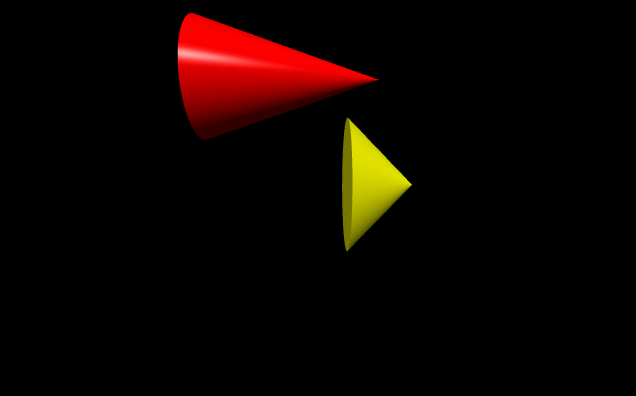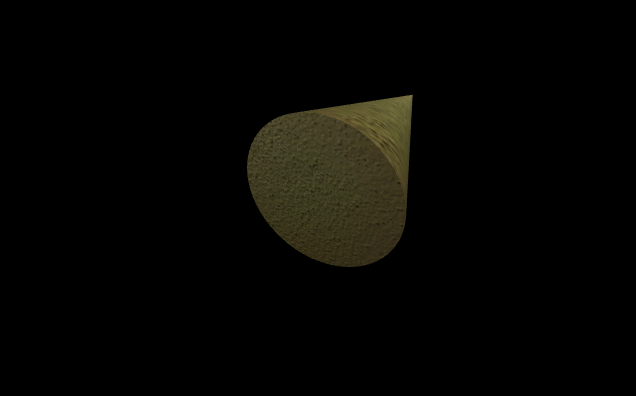# Making a cone with VPython

• Last Updated : 08 Jun, 2020

`VPython` makes it easy to create navigable 3D displays and animations, even for those with limited programming experience. Because it is based on Python, it also has much to offer for experienced programmers and researchers. `VPython` allows users to create objects such as spheres and cones in 3D space and displays these objects in a window. This makes it easy to create simple visualizations, allowing programmers to focus more on the computational aspect of their programs. The simplicity of `VPython` has made it a tool for the illustration of simple physics, especially in the educational environment.

Installation :

`pip install vpython`

A cone is a geometrical object in three-dimensional space that tapers smoothly from a flat circular base to a point called the apex or vertex. We can generate a cone in `VPython` using the `cone()` method.

## cone()

Syntax : cone(parameters)

Parameters :

• pos : It is the position of the center of the base of the cone. Assign a vector containing 3 values, example pos = vector(0, 0, 0)
• axis : It is the axis of alignment of the cone. Assign a vector containing 3 values, example axis = vector(1, 2, 1)
• up : It is the orientation of the cone. Assign a vector containing 3 values, example up = vector(0, 1, 0)
• color : It is the color of the cone. Assign a vector containing 3 values, example color = vector(1, 1, 1) will give the color white
• opacity : It is the opacity of the cone. Assign a floating value in which 1 is the most opaque and 0 the least opaque, example opacity = 0.5
• shininess : It is the shininess of the cone. Assign a floating value in which 1 is the most shiny and 0 the least shiny, example shininess = 0.6
• emissive : It is the emissivity of the cone. Assign a boolean value in which True is emissive and False is not emissive, example emissivity = False
• texture : It is the texture of the cone. Assign the required texture from the textures class, example texture = textures.stucco
• length : It is the length of the cone. Assign a floating value, the default length is 1, example length = 10
• radius : It is the radius of the base of the cone. Assign a floating value, the default height is 1, example radius = 5
• size : It is the size of the cone. Assign a vector containing 3 values representing the length, height and width respectively, example size = vector(1, 1, 1)

All the parameters are optional.

Example 1 :A cone with no parameters, all the parameters will have the default value.

 `# import the module``from` `vpython ``import` `*` `cone()`

Output :Example 2 :A cone using the parameters color, opacity, shininess and emissivity.

 `# import the module``from` `vpython ``import` `*` `cone(color ``=` `vector(``0``, ``1``, ``1``), ``     ``opacity ``=` `0.5``, ``     ``shininess ``=` `1``, ``     ``emissive ``=` `False``)`

Output :Example 3 :Displaying 2 cones to visualize the attributes pos, length and radius.

 `# import the module``from` `vpython ``import` `*`` ` `# the first cone``cone(pos ``=` `vector(``-``2``, ``2``, ``0``),``     ``length ``=` `3``,``     ``radius ``=` `1``,``     ``color ``=` `vector(``1``, ``0``, ``0``))`` ` `# the second cone``cone(pos ``=` `vector(``0.4``, ``0.2``, ``0.6``), ``     ``color ``=` `vector(``1``, ``1``, ``0``))`

Output :Example 4 :A cone using the parameters texture, axis and up.

 `# import the module``from` `vpython ``import` `*` `cone(texture ``=` `textures.stucco,``     ``axis ``=` `vector(``-``1``, ``4``, ``0``),``     ``up ``=` `vector(``1``, ``2``, ``2``))`

Output :My Personal Notes arrow_drop_up